Chapter 4 Part 2 - Income Determination and Multiplier

Economics Class 12
Macroeconomics

## What is Fixed Price Model?

As per this model,

It is assumed that in short run,

Prices of Commodity remain Fixed

Hence, Suppliers are willing to supply whatever amount consumers will demand at a constant price

So, Aggregate Supply remains constant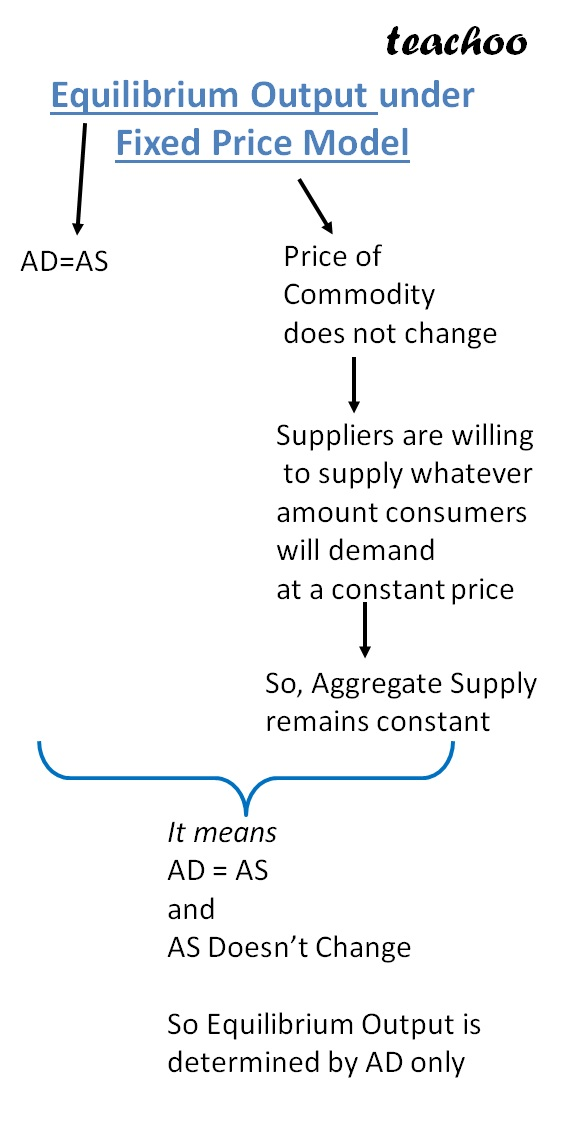## How to Determine equilibrium Output under Fixed Price Model

As per this model,

Since Aggregate Supply remains constant

Equilibrium Output of final gods is determined solely by Aggregate Demand

Now, we know that

Aggregate Demand = Consumption Expenditure + investment Expenditure

Note - This Investment Expenditure is Autonomous Expenditure

It does not vary with Income

So we can write it as

Also, we know that

Part of Consumption is Fixed and Part of consumption varies with Increase in Income

#### Equation of Consumption Function

it is represented by

C =Ĉ +b(Y)

where

C= Total Consumption

Ĉ = Autonomous Consumption

Y=Income

b=MPC

Hence, we have 2 equations

C =Ĉ +b(Y)

Putting the value of  in 

Here Ā is total Autonomous Expenditure

it is total of Autonomous Investment Expenditure + Autonomous Consumption Expenditure

Ā = Ĉ + Î

## SUMMARY

Now, we know that

equilibrium Point is determined at a point where

Now ,Value of AS Does not change (we follow fixed price model)

But Value of AD Can change (as it depends upon Autonomous Consumption as AD=Ā +b(Y)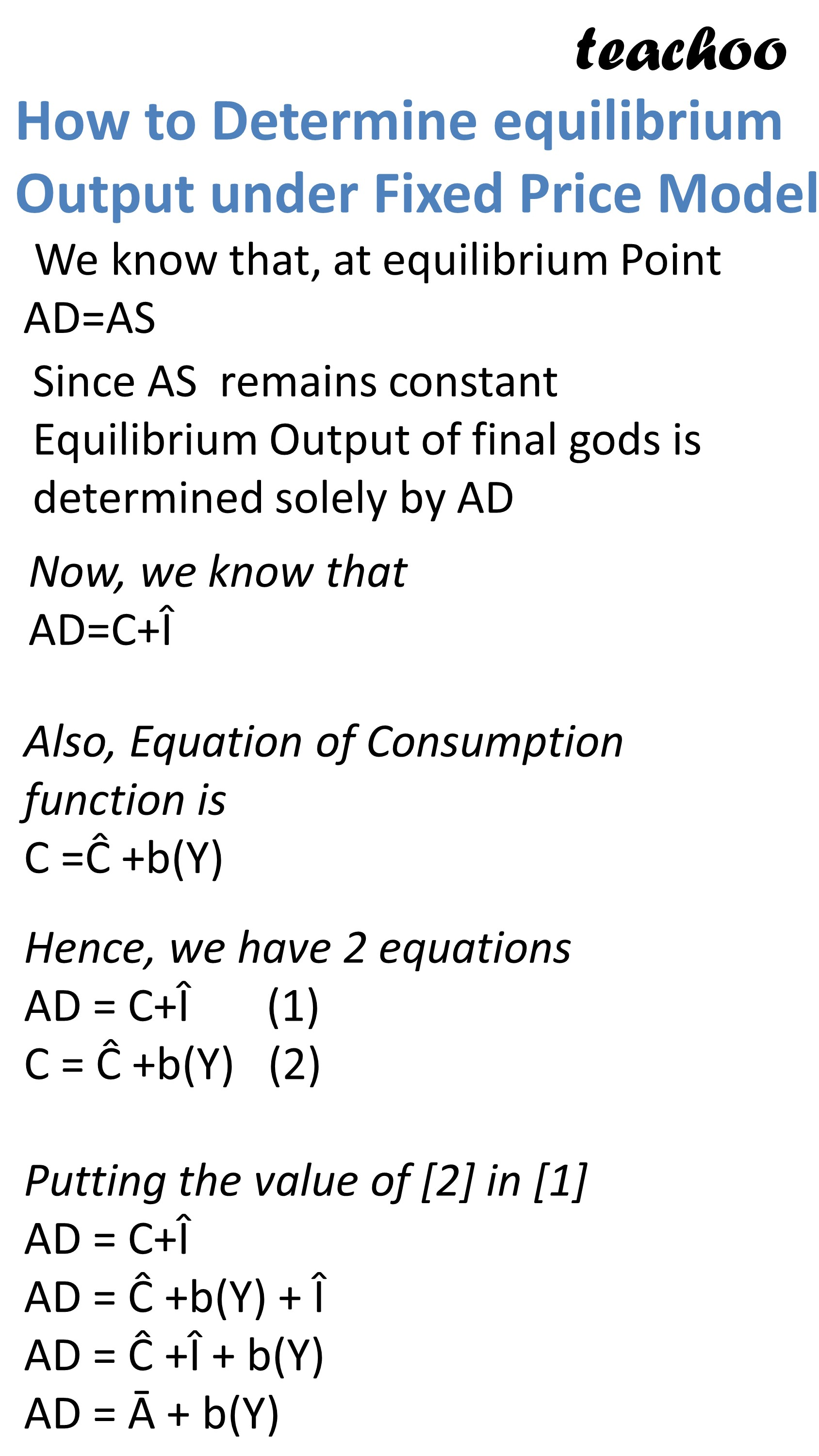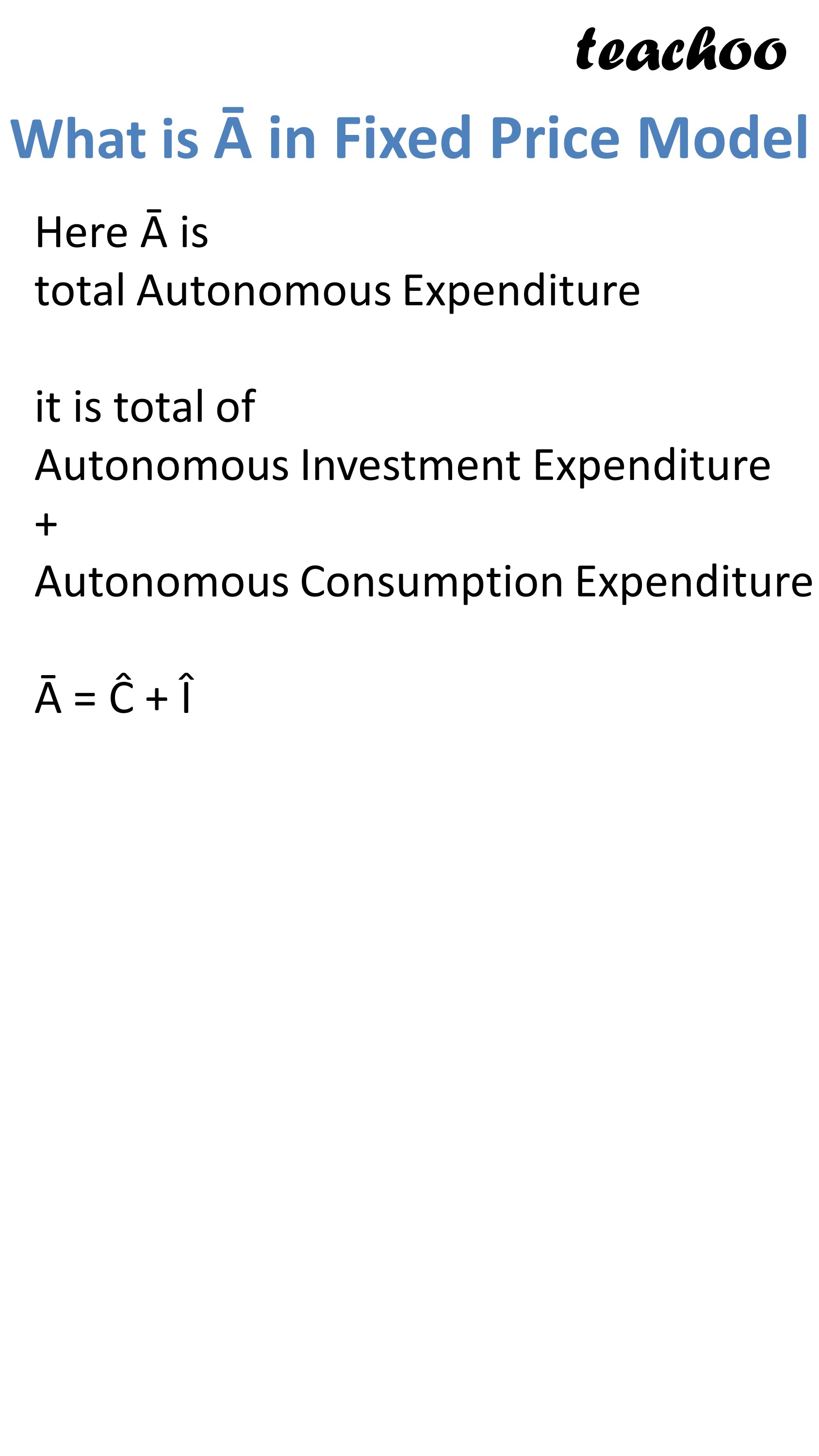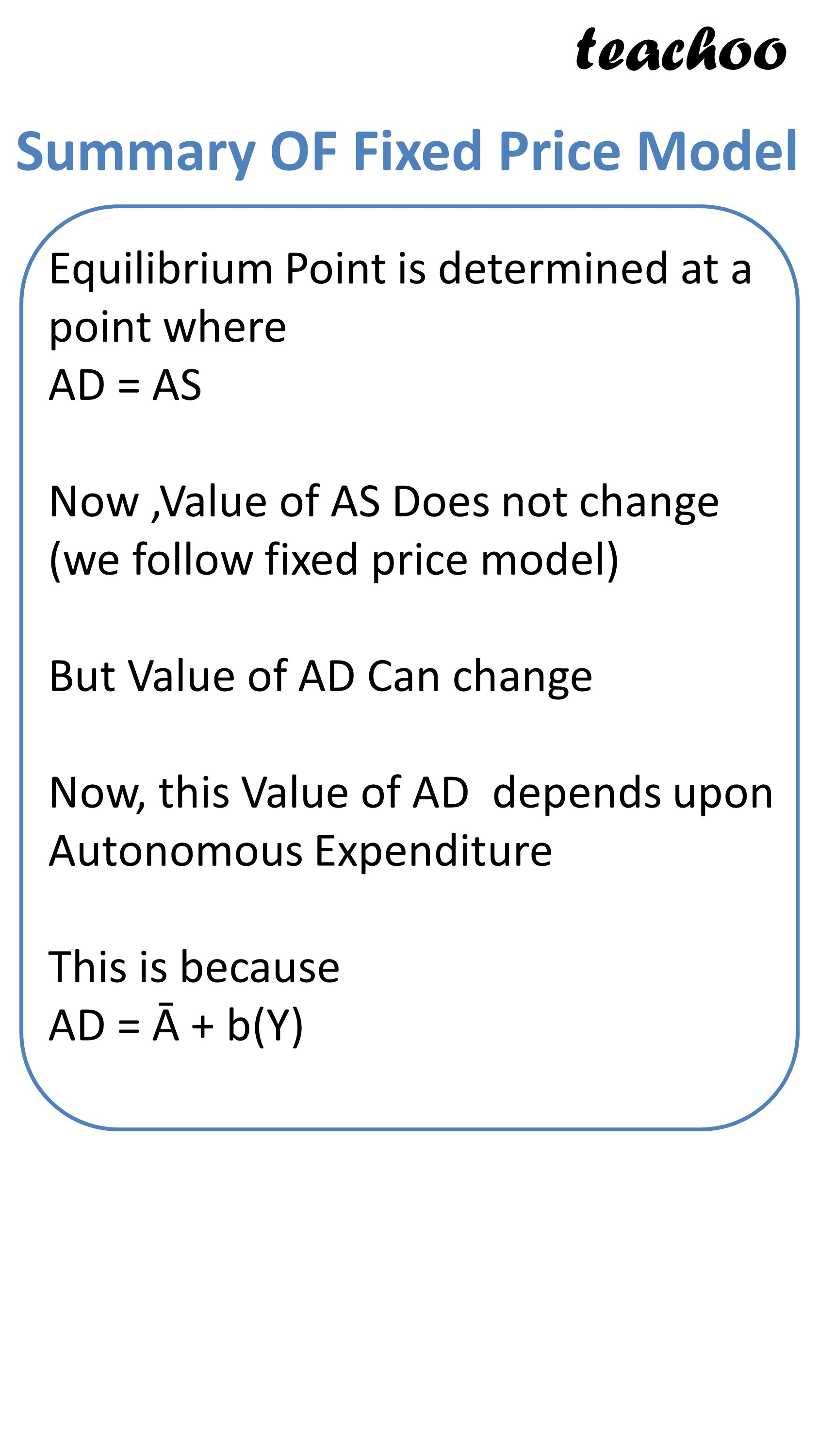## Graph Presentation

On X Axis, we represent Income or Output

On Y Axis, we show Demand

We draw AD curve starting from Point Ā1 (which is Autonomous Consumption)

Hence, equilibrium Point is determined at Point E where AD and AS Curves intersects

Now ,Value of AS Does not change (we follow fixed price model)

But Value of AD Can change (as it depends upon Autonomous Consumption as AD=Ā +b(Y)0

Suppose Autonomous Expenditure increases from Point Ā1 to Point Ā2,due to which AD Shifts to AD1

In this case, Equilibrium Point also changes from E to E1

Hence, Equilibrium Output also changes from OY to OY1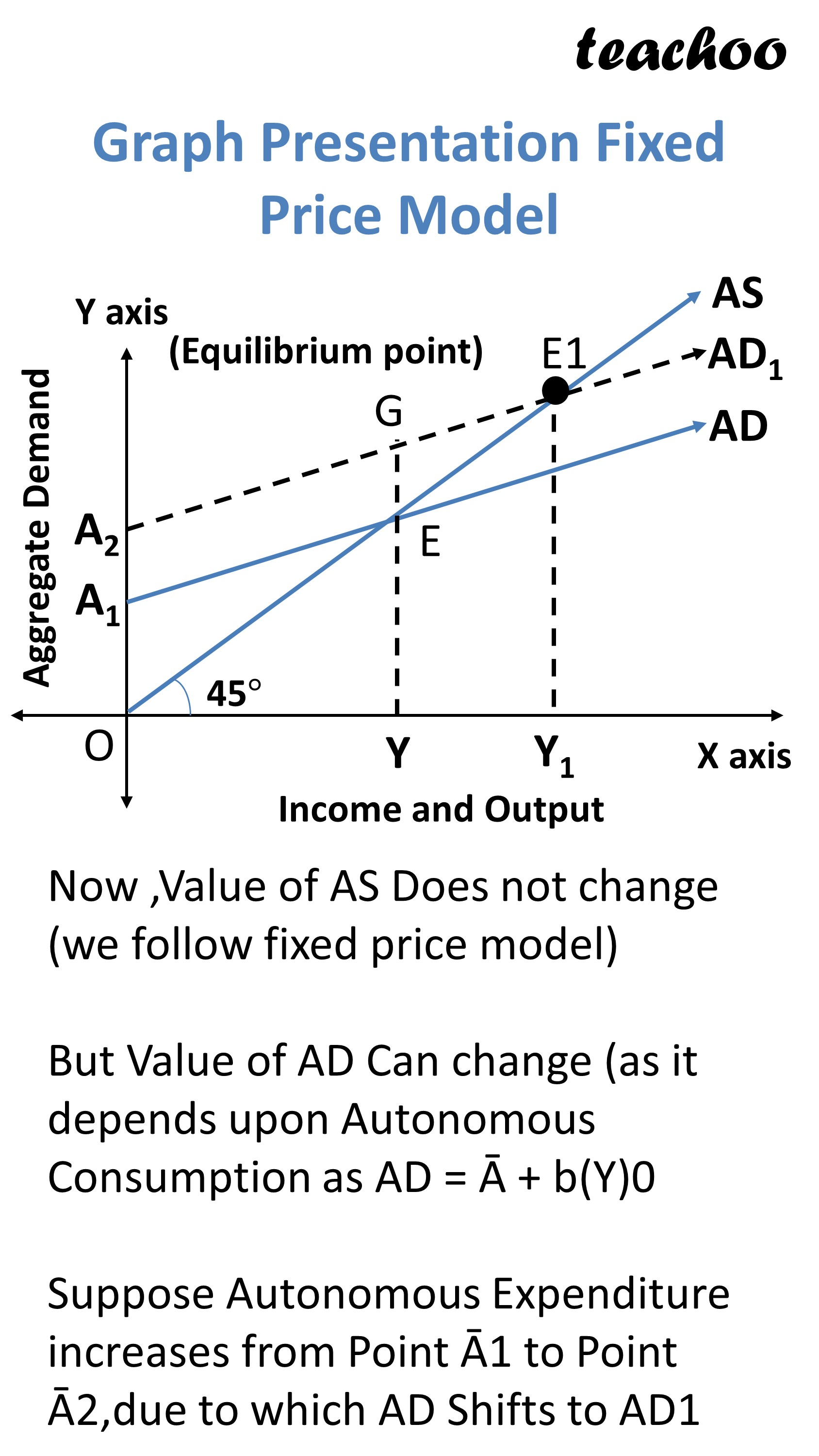Example Sandeep Garg Page 8.8

In this question, we are given

MPS=0.2

Income=Y=300 Crores

Also, total of Autonomous and Consumption Expenditure=50 Crores

It means

Ā =300 Crores

Now we know that

We are given value of A an Y

First we need to find b(MPC)

Step 1

WE KNOW THAT

MPC+MPS=1

MPC+0.2=1

MPC=1-0.2

MPC=0.8

b=0.8

Step 2

### NCERT Questions

#### Question 5

Measure the level of ex-ante aggregate demand when autonomous investment and consumption expenditure

(A) is Rs 50 crores, and MPS is 0.2 and level of income (Y) is Rs 4000 crores.

State whether the economy is in equilibrium or not (cite reasons).

### Other Books

#### Question 1

National Income = 1000

MPC = 0.7

Autonomous Consumption and Investment Expenditure = Ā = 200

Identify whether economy is in equilibrium or not?

Learn in your speed, with individual attention - Teachoo Maths 1-on-1 Class

### Transcript